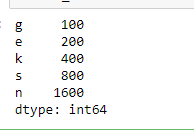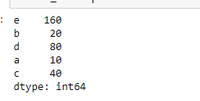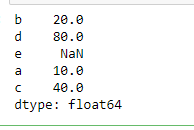GFG App
Open AppBrowser
Continue

# How to convert a dictionary to a Pandas series?

Let’s discuss how to convert a dictionary into pandas series in Python. A series is a one-dimensional labeled array which can contain any type of data i.e. integer, float, string, python objects, etc. while dictionary is an unordered collection of key : value pairs. We use series() function of pandas library to convert a dictionary into series by passing the dictionary as an argument.

Let’s see some examples:

Example 1: We pass the name of dictionary as an argument in series() function. The order of output will be same as of dictionary.

## Python3

 `# Import pandas library` `import` `pandas as pd`   `# Create a dictionary` `d ``=` `{``'g'` `: ``100``, ``'e'` `: ``200``,` `     ``'k'` `: ``400``, ``'s'` `: ``800``,` `     ``'n'` `: ``1600``}`   `# Convert from dictionary to series` `result_series ``=` `pd.Series(d)`   `# Print series` `result_series`

Output:Example 2: We pass the name of dictionary and a different order of index. The order of output will be same as the order we passed in the argument.

## Python3

 `# Import pandas library` `import` `pandas as pd`   `# Create a dictionary` `d ``=` `{``'a'` `: ``10``, ``'b'` `: ``20``, ` `     ``'c'` `: ``40``, ``'d'` `:``80``, ` `     ``'e'` `:``160``}`   ` `  `# Convert from dictionary to series` `result_series ``=` `pd.Series(d, index ``=` `[``'e'``, ``'b'``, ` `                                      ``'d'``, ``'a'``, ` `                                      ``'c'``])` `# Print series` `result_series`

Output:Example 3: In the above example the length of index list was same as the number of keys in the dictionary. What happens if they are not equal let’s see with the help of an example.

## Python3

 `# Import pandas library` `import` `pandas as pd`   `# Create a dictionary` `d ``=` `{``'a'` `: ``10``, ``'b'` `: ``20``, ` `     ``'c'` `: ``40``, ``'d'``:``80``}`   `# Convert from dictionary to series` `result_series ``=` `pd.Series(d, index ``=` `[``'b'``, ``'d'``,` `                                      ``'e'``, ``'a'``,` `                                      ``'c'``])` `# Print series` `result_series`

Output:So It’s assigning NaN value to that corresponding index.

My Personal Notes arrow_drop_up